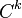# Real Lie group

This article gives a basic definition in the following area: Lie theory
View other basic definitions in Lie theory |View terms related to Lie theory |View facts related to Lie theory

This article describes a compatible combination of two structures: group and differential manifold

This article defines the notion of group object in the category of differential manifolds|View other types of group objects

## Definition

### General definition

A real Lie group is defined in the following equivalent ways as a group$G$ with any of the following equivalent pieces of additional structure information:

No. Name of additional structure:$G$ is a ... Compatibility condition on group multiplication Compatibility condition on inverse map
1 real-analytic manifold real-analytic map from$G \times G$ (with the product analytic manifold structure) to$G$ real-analytic map from$G$ to$G$.
2 differential manifold (in the$C^\infty$-sense)$C^\infty$-map (i.e., infinitely differentiable map) from$G \times G$ with the product manifold structure to$G$$C^\infty$-map from$G$ to$G$.
3$C^k$-manifold for some fixed positive$k$$C^k$-map (i.e.,$k$ times differentiable map) from$G \times G$ with the product manifold structure to$G$$C^k$-map from$G$ to$G$.
4 manifold in the topological sense continuous from$G \times G$ with the product topology to$G$ continuous from$G$ to$G$.

### Interpretation in the finite-dimensional and infinite-dimensional cases

The usual definitions of the terms real-analytic manifold, differential manifold, and manifold, rely on being finite-dimensional. However, all these notions have infinite-dimensional analogues that are, unfortunately, not unique. Depending on the context, the term real Lie group or Lie group may refer only to finite-dimensional real Lie group or it may refer to one of the broader definitions. The most common broader definition used is that of a real Banach Lie group.

### Equivalence of definitions

The definitions are equivalent because of the following two facts:

• Given any of the stronger structures, we can reduce to a weaker structure (The structures as listed above are from stronger to weaker: real-analytic to$C^\infty$ to$C^k$ to topological).
• In the reverse direction, for any choice of weaker structure, there is a unique choice of stronger structure such that the group operations continue to satisfy the compatibility conditions on the stronger structure.

The equivalence of definitions was proved in an attempt to resolve Hilbert's fifth problem.